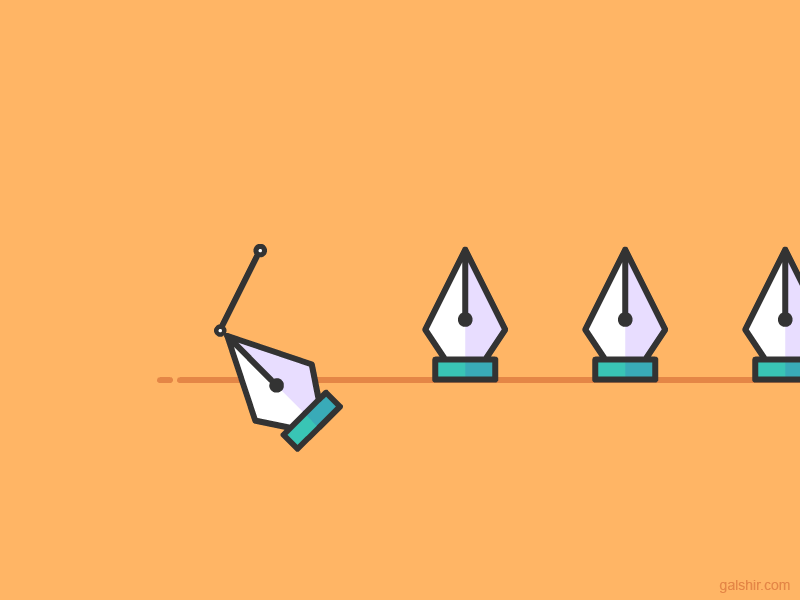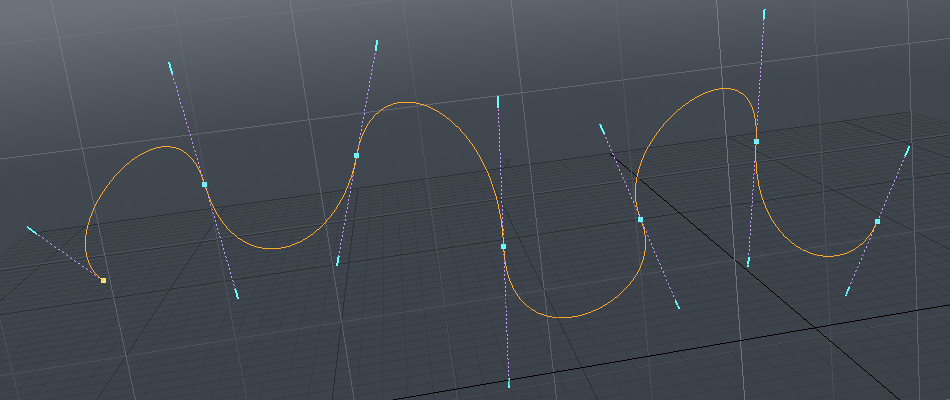I have always been fascinated by the simplicity and versatility of the pen tool in Adobe Illustrator.The tool support creation and editing of bézier curves using a simple user interaction. Illustrator support linear, quadratic and cubic bézier curves.

A straight line is a linear bézier curve.A line with one external point is quadratic bézier curve.A line with two external points is cubic bézier curve.The way Illustrator support creation and editing of bézier curves is to put a handle on each line end point. On a cubic curve one handle is located on point `P1` and one on point `P2`.A curve with a single handle represent a quadratic bézier curve and a line with no handles a linear.

First I would like to implement bézier curve approximation in Haskell.

The implementation is quite straight forward. The `bezier` function defines one basic implementation for a linear bézier curve.

Quadratic or higher bézier can be calculated by defining a new set of lines between the bézier points of each line segment and recursively calculating the bézier of that line set, until we only have a single line left. See animations above.

For instance for a cubic bézier you have 3 line segments (P01, P12, P23). Two lines is defined by connecting the bézier points on these three lines which is used to calculate a quadratic bézier. The quadratic bézier can in the same way be reduced to a linear (which we have implemented).

``````module Main where

import Graphics.Gloss
import qualified Graphics.Gloss.Data.Point.Arithmetic as G
import Graphics.Gloss.Data.Vector
import Prelude hiding (lines)

main :: IO ()
main = do display (InWindow "Bezier" (400, 400) (20, 20)) black \$ pic

type Bezier = [Point]

pairs :: [a] -> [(a,a)]
pairs xs = zip xs (drop 1 xs)

-- Generalized higher order Bézier approximation in a single point u [0..1]
bezier :: Bezier -> Float -> Point
bezier (p1:p2:[]) u = p1 G.+ (u G.* (p2 G.- p1))                            -- linear
bezier ps         u = let ps' = fmap (\(a,b) -> bezier [a,b] u) \$ pairs ps  -- quadratic or higher
in  bezier ps' u

-- Draw approximate Bézier
renderBezier :: Bezier -> Picture
renderBezier b = let len = foldl (\s (a,b) -> s + magV (b G.- a)) 0 \$ pairs b
num = floor (len / 5)      -- resolution about 5 pixels
steps = fmap ((*) (1/(fromIntegral num)) . fromIntegral) [0..num]
in line \$ fmap (bezier b) steps

-- Draw Bézier including control points
showBezier :: Bezier -> Picture
showBezier b = pictures [ Color green \$ renderBezier b          -- curve
, Color orange \$ line [head b, last b]  -- linear
, Color (greyN 0.75) \$ line b]          -- control points

pic :: Picture
pic = Color white
\$ showBezier [(0,0),(0,100),(250,200),(200,0)]

``````

The resulting screenshot can be found below.## UI for manipulating Bézier curves

The following table summarize a minimal set of actions to allow creation and modification of Bézier curves.

``````State       | Mouse Loc.   | Action            | Description                    | New state
----------- | ------------ | ----------------- | ------------------------------ | ---------
Select      | Empty space  | Click             | Start path with (no handles)   | Draw
"           | Empty space  | Click-drag        | Start path (handles)           | Draw
Draw        | Empty space  | Click             | Add point to path (no handles) | -
"           | Empty space  | Click-drag        | Add point to path (handles)    | -
"           | -            | Escape            | Undo last edit                 | -
Draw/Select | Handle       | Click-drag        | Modify handles on path [^1]    | -
"           | Handle       | Alt + Click-drag  | Modify single handle on path   | -
"           | Point        | Click-drag        | Modify point on path           | -
Draw        | Empty space  | Right-Click       | Finish path                    | Select
Select      | Point        | Alt + Click       | Toggle point in selection      | -
"           | Point        | Alt2 + Click-drag | Add handles to point           | -
"           | Path segmemt | Alt + Click       | Toggle path in selection       | -
"           | Path segmemt | Alt2 + Click      | Insert/remove point on path    | -
"           | Selection    | Click-drag        | Move selection                 | -
"           | Empty space  | Click             | Clear selection                | -
"           | -            | Delete            | Delete selection               | -

[^1]: Handle may be dropped on a point to remove the handle

TODO: Support square select/deselect in Select mode
``````

See dotgrid and ronin for interesting implementations.